# SAT II Math II : Surface Area

## Example Questions

### Example Question #1 : Surface Area

A rectangular swimming pool ismeters deep throughout and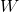meters wide. Its length is ten meters greater than twice its width. Which of the following expressions gives the total surface area, in square meters, of the inside of the pool?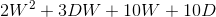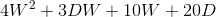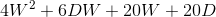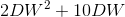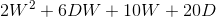Explanation:

Since the length of the pool is ten meters longer than twice its width, its length is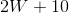.

The inside of the pool can be seen as a rectangular prism. The bottom, or the base, has dimensionsand, so its area is the product of these: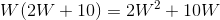The sides of the pool have depth. Two sides have widthand therefore have area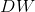.

Two sides have widthand therefore have area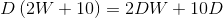The total area of the inside of the pool is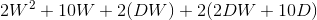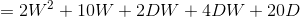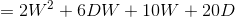### Example Question #2 : Surface Area

A water tank takes the shape of a closed rectangular prism whose exterior has height 30 feet, length 20 feet, and width 15 feet. Its walls are one foot thick throughout. What is the total surface area of the interior of the tank?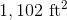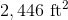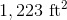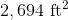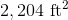Explanation:

The height,  length, and width of the interior tank are each two feet less than the corresponding dimension of the exterior of the tank, so the dimensions of the interior are 28, 18, and 13 feet. The surface area of the interior is what we are looking for here. It comprises six rectangles:

Two with area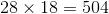square feet;

Two with area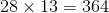square feet;

Two with area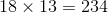square feet.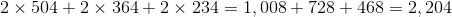square feet.

### Example Question #3 : Surface Area

What is the surface area of a cube with a side length of 5?Explanation:

If you were to take apart a cube so that you could lay it flat on a surface, you would be able to see that a cube is just made up of 6 identical squares. The area of one square is the length of the side squared, so the surface area of the cube would be denoted with the formula: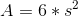In this case the side length is 5, so plugging that into the formula will get the answer.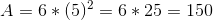### Example Question #4 : Surface Area

Find the surface area of a rectangular prism with length, width, and height dimensions of, and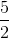, respectively.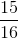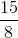Explanation:

Be careful not to confuse surface area with volume!

There are 6 faces in a rectangular prism, and we will need the sum of all the areas of each face.

Write the formula.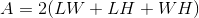Substitute the dimensions.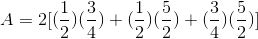Evaluate each product in the bracket.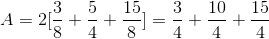Combine the terms of the numerator.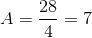The answer is:### Example Question #5 : Surface Area

What is the surface area of a cube with a side length of?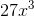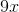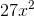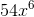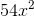Explanation:

Write the formula for the surface area of a cube.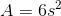Substitute the side length.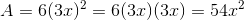The answer is:### Example Question #6 : Surface Area

Find the surface area of a sphere with a radius of 3.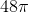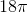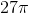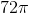Explanation:

Write the formula for the surface area of a sphere.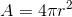Substitute the radius into the equation.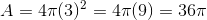The answer is:### Example Question #7 : Surface Area

Find the surface area of a sphere with a radius of 2.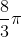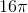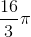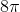Explanation:

Write the formula for the surface area of a sphere.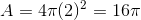The answer is:### Example Question #411 : Sat Subject Test In Math Ii

Find the surface area of a cube if the area of one of the square faces is.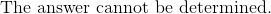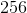Explanation:

Write the formula for the surface area of a cube.The area of a square side is already given.  Substitute that as the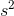term.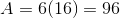The answer is: